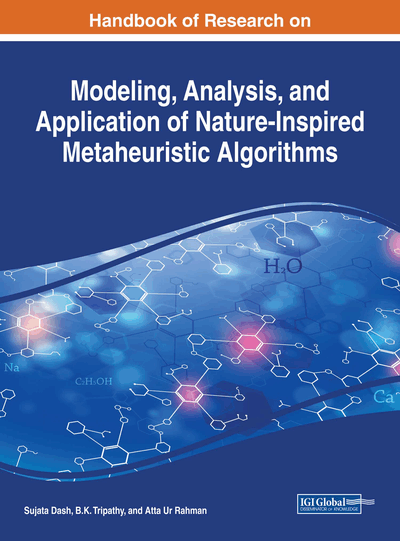# A Holistic-Based Multi-Criterion Decision-Making Approach for Solving Engineering Sciences Problem Under Imprecise Environment

Syed Abou Iltaf Hussain (NIT Agartala, India), Sankar Prasad Mondal (Midnapore College (Autonomous), India) and Uttam Kumar Mandal (NIT Agartala, India)
DOI: 10.4018/978-1-5225-2857-9.ch015
Available
\$37.50
No Current Special Offers

## Abstract

Multi-Criteria Decision Making has evolved as an important tool for taking some of the most important decisions in the today's hi-tech engineering world. But due to some reasons like measurement difficulty, lack of data, faulty instruments, etc., or due to lack of absolute information about the topic, alternatives present and the criteria, decision making becomes very difficult as all parameter for modeling a decision making problem are not precise. In such scenario the importance of one with respect to the others are represented in terms of linguistic factor. Such cases could be tackled by considering the problem in fuzzy environment. In this chapter, the different hybrid fuzzy MCDM techniques are shown along with their application in different engineering problems. One problem is randomly selected and solved using different fuzzy MCDM techniques and compared the result with the existing literature.
Chapter Preview
Top

## 1. Introduction

### 1.1. Fuzzy MCDM

In engineering, MCDM has become one of the major tools of decision making in a scenario where decision makers have to take a decision which is guided by various criteria. Due to different reasons some known and some unknown, modelling by MCDM for decision making problem are not precise. One of the major reasons for this impreciseness is the lack of concrete rule for converting linguistic terms into quantitative values. In such case we can take the help of fuzzy set theory. Hybrid fuzzy-MCDM models are applied in different engineering fields such as reliability engineering, robotics, scheduling, manufacturing, etc. (Kumar and Gag, 2010; KeshavarzGhorabaee et al., 2015a, 2015c; Amiri et al., 2014), machine selection (Kemal Vatansever and YiğitKazançoğlu, 2014), selection of machining tools etc.

### 1.2. Motivation of the Chapter

Decision making theory is very important for engineering science problem. When some complication comes to some model or problem, then there we have to take multi criteria decision, in other words the problem belongs to MCDM problem. For practical point of view some of the parameter or some conditions may not be precisely known. For that cases, we have to consider impreciseness. Fuzzy set theory is one of the tool to define such impreciseness. Our main aim is to discuss how MCDM problem behave in fuzzy environment, also how can we solve the problem. In engineering sciences, the fuzzy MCDM problem play an important role, how it works.

### 1.3. Novelties

A lot of works on fuzzy MCDM have been done over the globe by different researchers and scientists. Yet a lot of work still has to be done in this field. In our chapter, we try to find some new links and some new result, comparison of results with different examples is as follows:

• 1.

How fuzzy set theory are considered for measuring the uncertainty.

• 2.

How MCDM problem is different with fuzzy MCDM problem.

• 3.

Comparison between different fuzzy MCDM techniques.

• 4.

Application of fuzzy MCDM techniques in engineering sciences.

### 1.4. Structure of the Chapter

The organization of the paper is as follows. In the first section, it is an introduction to the chapter followed by motivation which led to the selection of the particular topic and novelties. The second section consists of basic concept of the MCDM and fuzzy set theory and their application in the engineering science problem. The second section also contains different types of environment. Section 3 and section 4 contains different types of fuzzy MCDM models and the models are used for solving a problem respectively which is further compared with the existing literature in section 4. Section 5 is the conclusion part of the chapter.

Top

## 2. Preliminaries

### 2.1. Basic Concept of MCDM

• Definition 2.1.a: Decision is defined as the final submission or the conclusion reached after consideration.

• Definition 2.1.b: In cognitive science, selection of an action or a belief from a set of alternatives is called Decision Making.

Selecting an action from a set of alternatives by considering various criteria effecting the judgement either to the same or different extent is called Multi-Criteria Decision Making. In MCDM, set of alternatives are the available possibilities or the choices from which the best possibility is to be selected by considering different factors influencing the outcome. Ranking of the alternative is done in ascending order starting from the best to the worst alternative. The alternatives are ranked in on the basis of some factors influencing the results. These factors are called Criteria. Criterion is defined as the factors on the basis of which a decision is taken. In MCDM, there is a number of criterion. Each criterion effects the final judgement either to the same or different extent.

## Complete Chapter List

Search this Book:
Reset